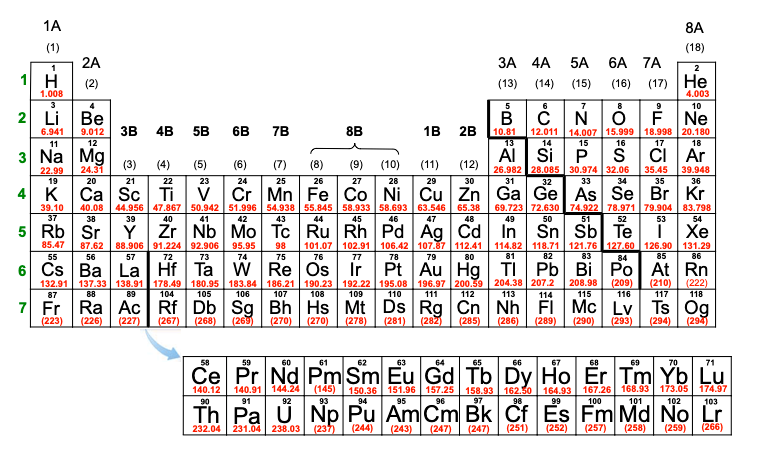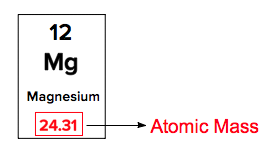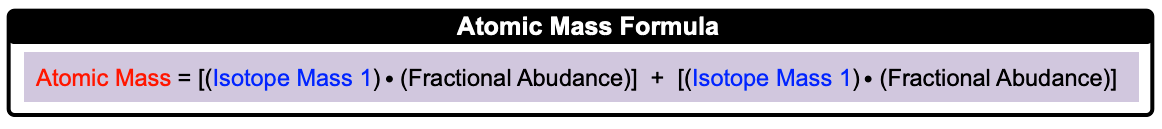Clutch Prep is now a part of Pearson
Ch.2 - Atoms & ElementsWorksheetSee all chapters

# Atomic Mass

See all sections
Sections
The Atom
Subatomic Particles
Isotopes
Ions
Atomic Mass
Periodic Table: Classifications
Periodic Table: Group Names
Periodic Table: Representative Elements & Transition Metals
Periodic Table: Element Symbols
Periodic Table: Elemental Forms
Periodic Table: Phases
Periodic Table: Charges
Calculating Molar Mass
Mole Concept
Law of Conservation of Mass
Law of Definite Proportions
Atomic Theory
Law of Multiple Proportions
Millikan Oil Drop Experiment
Rutherford Gold Foil Experiment

The atomic mass of an element includes the masses of all 3 subatomic particles of neutrons, protons and electrons.

###### Determining Atomic Mass

Concept #1: The atomic masses of elements can be found on the Periodic Table.Example #1: Which of the following represents an element from the first column with the greatest atomic mass?

On the Periodic Table, the atomic mass is represented by the number with decimal places.Practice: Which of the following choices has the greatest atomic mass?

Concept #2: Calculating Atomic MassExample #2: Calculate the atomic mass of gallium if gallium has 2 naturally occurring isotopes with the following masses and natural abundances:

Ga-69          68.9256 amu          60.11%
Ga-71           70.9247 amu           39.89%

Concept #3: Calculating Atomic Mass (No Fractional Abundances)

Example #3: Boron has two naturally occurring isotopes, 10B has a mass of 10.013 amu and 11B has a mass of 11.009 amu. What is the natural or % abundance of the 10B isotope?

Practice: Only three isotopes of magnesium exist on earth. 24Mg is the most common form at 78.70% natural abundance with a mass of 23.98504 amu, 25Mg has a 10.13% natural abundance, while 26Mg has a natural abundance of 11.17% and a mass of 25.98259 amu. What is the mass of the 25Mg isotope?

Practice: Silver has an atomic mass of 107.868 amu. The Ag-109 isotope (108.905 amu) is 48.16%. What is the amu of the other isotope?